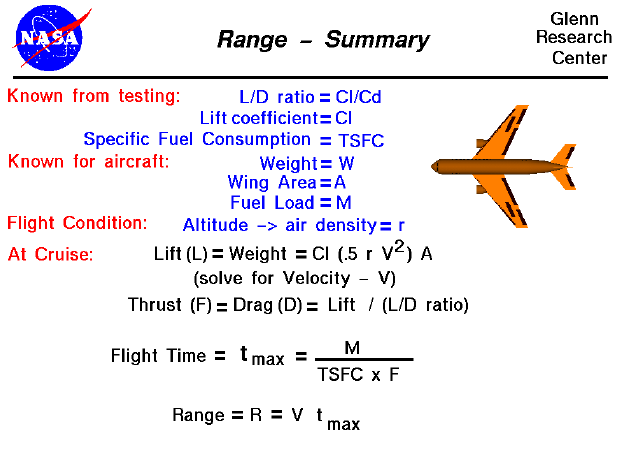An interactive Java applet which demonstrates the information found on this slide is also available. This applet presents problems which you must solve by using the range equation.

On this page we summarize the information necessary to do a preliminary calculation of an aircraft's range under cruise conditions. On this page, we are taking a very simple view of aircraft range - for academic purposes. In reality, calculating the range is a complex problem because of the large number of variables. An aircraft's flight is not conducted at a single ground speed but varies from zero (at take-off) to cruise conditions and back to zero (at landing). Extra fuel is expended in climbing to altitude and in maneuvering the aircraft. The weight is constantly changing as fuel is burned, so the lift, drag, and thrust (fuel consumption rate) are also continually changing. On real aircraft, just like with your automobile, there is usually a fuel reserve and the pilot makes sure to land the plane with fuel still on board. We are going to neglect all of these effects.

There are certain things that we need to know about our aircraft. From previous wind tunnel testing, we need to determine the L/D ratio (Cl/Cd) and the lift coefficient (Cl). We also need some information about the propulsion system, specifically, the specific fuel consumption rate (TSFC) of the engine. For the aircraft itself, we need to know the weight (W) of the aircraft, the wing area, (A) and the fuel load (M). We are free to select a flight altitude, but this determines the air density (r) from our model of the atmosphere.

In cruise, the lift (L) is equal to the weight (W) and the thrust (F) is equal to the drag (D). Using the lift equation we can solve for the velocity necessary to create enough lift to equal the weight.

L = W = Cl * .5 * r * V ^2 * A

In this equation, all of the variables are known except the velocity (V), so we solve this equation for V. Using the L/D ratio, we can solve for the drag of the aircraft which is equal to the thrust.

F = D = L / (Cl/Cd)

The thrust, specific fuel consumption, and fuel load determine the maximum flight time available to the aircraft.

t max = M / (TSFC * F)

This flight time multiplied by the aircraft velocity determines the range.

range = V * t maxGuided Tours